### Matrix Addition, Subtraction, Multiplication and transpose in java

Contents of page >
1) Matrix Addition in java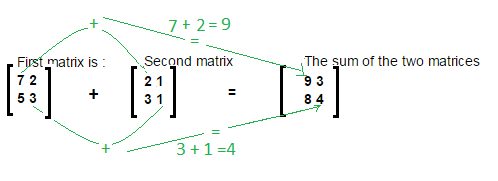2) Matrix Subtraction in java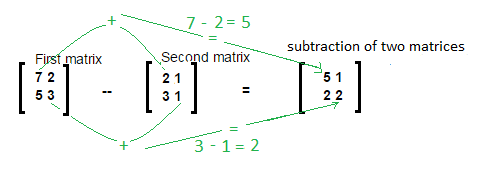3) Matrix Multiplication in java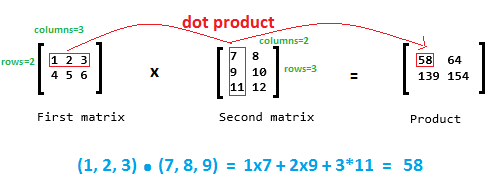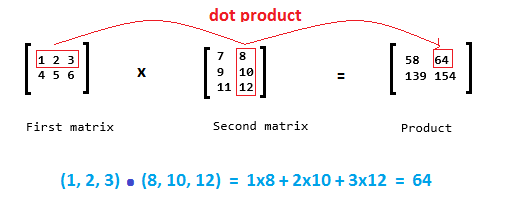4) Matrix Transpose in java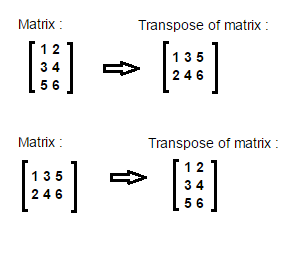Hi! In this core java programming tutorial will learn how to add two matrices in java.
Both matrices must have same number of rows and columns in java.

Let’s understand addition of matrices by diagram.//Subtraction of matrices.
int[][] resultMatix = new int[rows][columns];
for (int i = 0; i < rows; i++) {
for (int j = 0; j < columns; j++) {
resultMatix[i][j] = matrix1[i][j] + matrix2[i][j];
}
}

Example/Full Program/SourceCode in java>
 package matrix; import java.util.Scanner; /** * @author AnkitMittal */ /** Copyright (c), AnkitMittal  JavaMadeSoEasy.com */ public class MatrixAddition {    public static void main(String...args) {           Scanner scanner = new Scanner(System.in);           System.out.print("Enter number of rows in matrix : "); //rows and columns in matrix1 and matrix2 must be same for addition.           int rows = scanner.nextInt();           System.out.print("Enter number of columns in matrix : ");           int columns = scanner.nextInt();           int[][] matrix1 = new int[rows][columns];           int[][] matrix2 = new int[rows][columns];                     System.out.println("Enter the elements in first matrix :");           for (int i = 0; i < rows; i++) {                  for (int j = 0; j < columns; j++) {                        matrix1[i][j] = scanner.nextInt();                  }           }           System.out.println("Enter the elements in second matrix :");           for (int i = 0; i < rows; i++) {                  for (int j = 0; j < columns; j++) {                        matrix2[i][j] = scanner.nextInt();                  }           }                     //addition of matrices.           int[][] resultMatix = new int[rows][columns];           for (int i = 0; i < rows; i++) {                  for (int j = 0; j < columns; j++) {                        resultMatix[i][j] = matrix1[i][j] + matrix2[i][j];                  }           }           System.out.println("\nFirst matrix is : ");           for (int i = 0; i < rows; i++) {                  for (int j = 0; j < columns; j++) {                        System.out.print(matrix1[i][j] + " ");                  }                  System.out.println();           }           System.out.println("\nSecond matrix is : ");           for (int i = 0; i < rows; i++) {                  for (int j = 0; j < columns; j++) {                        System.out.print(matrix2[i][j] + " ");                  }                  System.out.println();           }           System.out.println("\nThe sum of the two matrices is : ");           for (int i = 0; i < rows; i++) {                  for (int j = 0; j < columns; j++) {                        System.out.print(resultMatix[i][j] + " ");                  }                  System.out.println();           }    } } /*OUTPUT Enter number of rows in matrix : 2 Enter number of columns in matrix : 2 Enter the elements in first matrix : 7 2 5 3 Enter the elements in second matrix : 2 1 3 1 First matrix is : 7 2 5 3 Second matrix is : 2 1 3 1 The sum of the two matrices is : 9 3 8 4 */

2) Matrix Subtraction in java

Hi! we will learn how to add subtract matrices in java.
Both matrices must have same number of rows and columns in java.

Let’s understand subtraction of matrices by diagram.Main logic behind subtraction in java is:
//Subtraction of matrices.
int[][] resultMatix = new int[rows][columns];
for (int i = 0; i < rows; i++) {
for (int j = 0; j < columns; j++) {
resultMatix[i][j] = matrix1[i][j] - matrix2[i][j];
}
}

Example/Full Program/SourceCode  in java>
 package matrix; import java.util.Scanner; /** * @author AnkitMittal */ /** Copyright (c), AnkitMittal  JavaMadeSoEasy.com */ public class MatrixSubtraction {    public static void main(String...args) {           Scanner scanner = new Scanner(System.in);           System.out.print("Enter number of rows in matrix : "); //rows and columns in matrix1 and matrix2 must be same for subtraction.           int rows = scanner.nextInt();           System.out.print("Enter number of columns in matrix : ");           int columns = scanner.nextInt();           int[][] matrix1 = new int[rows][columns];           int[][] matrix2 = new int[rows][columns];                     System.out.println("Enter the elements in first matrix :");           for (int i = 0; i < rows; i++) {                  for (int j = 0; j < columns; j++) {                        matrix1[i][j] = scanner.nextInt();                  }           }           System.out.println("Enter the elements in second matrix :");           for (int i = 0; i < rows; i++) {                  for (int j = 0; j < columns; j++) {                        matrix2[i][j] = scanner.nextInt();                  }           }                     //Subtraction of matrices.           int[][] resultMatix = new int[rows][columns];           for (int i = 0; i < rows; i++) {                  for (int j = 0; j < columns; j++) {                        resultMatix[i][j] = matrix1[i][j] - matrix2[i][j];                  }           }           System.out.println("\nFirst matrix is : ");           for (int i = 0; i < rows; i++) {                  for (int j = 0; j < columns; j++) {                        System.out.print(matrix1[i][j] + " ");                  }                  System.out.println();           }           System.out.println("\nSecond matrix is : ");           for (int i = 0; i < rows; i++) {                  for (int j = 0; j < columns; j++) {                        System.out.print(matrix2[i][j] + " ");                  }                  System.out.println();           }           System.out.println("\nThe subtraction of the two matrices is : ");           for (int i = 0; i < rows; i++) {                  for (int j = 0; j < columns; j++) {                        System.out.print(resultMatix[i][j] + " ");                  }                  System.out.println();           }    } } /*OUTPUT Enter number of rows in matrix : 2 Enter number of columns in matrix : 2 Enter the elements in first matrix : 7 2 5 3 Enter the elements in second matrix : 2 1 3 1 First matrix is : 7 2 5 3 Second matrix is : 2 1 3 1 The subtraction of the two matrices is : 5 1 2 2 */

3) Matrix Multiplication in java
Write a program to multiply matrix in java.

In multiplication columns in matrix1 must be equal to rows in matrix2

Let’s understand multiplication of matrices by diagram-
we will find out dot product.Main logic behind multiplication in java is:

//Multiply matrices
int[][] productMatrix  = new int[rowsMatrix1][columnsMatrix2];
for (int i = 0; i < rowsMatrix1; i++) {
for (int j = 0; j < columnsMatrix2; j++) {
for (int k = 0; k < columnsMatrix1RowsMatrix2; k++) { //columns in matrix1= rows in matrix2
productMatrix[i][j]= productMatrix[i][j] + matrix1[i][k] *matrix2[k][j];
}
}
}

Example / Full Program/SourceCode in java >
 package matrix; import java.util.Scanner; /** Copyright (c), AnkitMittal  JavaMadeSoEasy.com */ public class MatrixMultiplication {      public static void main(String...args) {       Scanner scanner = new Scanner(System.in);             System.out.print("Enter number of rows in first matrix : ");       int rowsMatrix1 = scanner.nextInt();       System.out.print("Enter number of columns in first matrix / rows in matrix2: ");       int columnsMatrix1RowsMatrix2 = scanner.nextInt();         //variable name used for understanding convenience, because columns in matrix1 = rows in matrix2       System.out.print("Enter number of columns in second matrix : ");       int columnsMatrix2 = scanner.nextInt();             int[][] matrix1 = new int[rowsMatrix1][columnsMatrix1RowsMatrix2];       int[][] matrix2 = new int[columnsMatrix1RowsMatrix2][columnsMatrix2];       System.out.println("Enter the first matrix in elements :");       for (int i = 0; i < matrix1.length; i++) {           for (int j = 0; j < matrix1.length; j++) {               matrix1[i][j] = scanner.nextInt();           }       }             System.out.println("Enter the second matrix elements:");       for (int i = 0; i < matrix2.length; i++) {           for (int j = 0; j < matrix2.length; j++) {               matrix2[i][j] = scanner.nextInt();           }       }                   //Multiply matrices       int[][] productMatrix  = new int[rowsMatrix1][columnsMatrix2];       for (int i = 0; i < rowsMatrix1; i++) {           for (int j = 0; j < columnsMatrix2; j++) {               for (int k = 0; k < columnsMatrix1RowsMatrix2; k++) { //columns in matrix1= rows in matrix2                  productMatrix[i][j] = productMatrix[i][j] + matrix1[i][k] * matrix2[k][j];               }           }       }                        System.out.println("\nFirst matrix is : ");                  for (int i = 0; i < rowsMatrix1; i++) {                        for (int j = 0; j < columnsMatrix1RowsMatrix2; j++) {                               System.out.print(matrix1[i][j] + " ");                        }                        System.out.println();                  }                  System.out.println("\nSecond matrix is : ");                  for (int i = 0; i < columnsMatrix1RowsMatrix2; i++) {                        for (int j = 0; j < columnsMatrix2; j++) {                               System.out.print(matrix2[i][j] + " ");                        }                        System.out.println();                  }                        System.out.println("\nProduct of matrix1 and matrix2 is");       for (int i = 0; i < rowsMatrix1; i++) {           for (int j = 0; j < columnsMatrix2; j++) {               System.out.print(productMatrix[i][j] + " ");           }           System.out.println();       }      }    } /*OUTPUT Enter number of rows in first matrix : 2 Enter number of columns in first matrix / rows in matrix2: 3 Enter number of columns in second matrix : 2 Enter the first matrix in elements : 1 2 3 4 5 6 Enter the second matrix elements: 7 8 9 10 11 12 First matrix is : 1 2 3 4 5 6 Second matrix is : 7 8 9 10 11 12 Product of matrix1 and matrix2 is: 58 64 139 154 */

4) Matrix Transpose in java
Write a program to transpose a matrix in java.Main logic behind transpose in java is:
//transpose matrix
int transpose[][] = new int[columns][rows];
for (int i = 0; i < rows; i++) {
for (int j = 0; j < columns; j++)
transpose[j][i] = matrix[i][j];
}

Example / Full Program/SourceCode in java >
 package matrix; import java.util.Scanner; /** Copyright (c), AnkitMittal  JavaMadeSoEasy.com */ public class TransposeMatrix {    public static void main(String...args) {           Scanner scanner = new Scanner(System.in);           System.out.println("Enter number of rows in matrix : ");           int rows = scanner.nextInt();           System.out.print("Enter number of columns in matrix : ");           int columns = scanner.nextInt();           int matrix[][] = new int[rows][columns];           System.out.println("Enter the elements in matrix :");           for (int i = 0; i < rows; i++) {                  for (int j = 0; j < columns; j++) {                        matrix[i][j] = scanner.nextInt();                  }           }                     //transpose matrix           int transpose[][] = new int[columns][rows];           for (int i = 0; i < rows; i++) {                  for (int j = 0; j < columns; j++)                        transpose[j][i] = matrix[i][j];           }           System.out.println("\nEntered Matrix is : ");           for (int i = 0; i < rows; i++) {                  for (int j = 0; j < columns; j++) {                        System.out.print(matrix[i][j] + " ");                  }                  System.out.println();           }                     System.out.println("\nTranspose of entered matrix is : ");           for (int i = 0; i < columns; i++) {                  for (int j = 0; j < rows; j++)                        System.out.print(transpose[i][j] + " ");                  System.out.println();           }    } } /*OUTPUT Enter number of rows in matrix : 3 Enter number of columns in matrix : 2 Enter the elements in matrix : 1 2 3 4 5 6 Entered Matrix is : 1 2 3 4 5 6 Transpose of entered matrix is : 1 3 5 2 4 6 */

Having any doubt? or you you liked the tutorial! Please comment in below section.